# How to denote integer number(ℤ) symbol in LaTeX?

Mathematically, set of integer numbers are denoted by blackboard-bold() form of “Z”. And the letter “Z” comes from the German word Zahlen(numbers).

Blackboard-bold is a style used to denote various mathematical symbols. For example natural numbers, real numbers, whole numbers, etc.

In latex, the \mathbb command is used to convert a latter to blackboard-bold form, and the latter is passed as an argument in the command.

And this \mathbb command is included in more than one package. For example

 amsfonts \mathbb{Z} →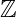amssymb \mathbb{Z} →txfonts \mathbb{Z} →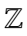pxfonts \mathbb{Z} →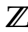\documentclass{article}
\usepackage{amsfonts}
\begin{document}
$\mathbb{Z}\subset\mathbb{Q}$
$\mathbb{Z}=\{\ldots,-2,-1,0,1,2,\ldots\}$
\end{document}

Output :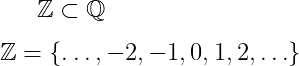Integer number sets are divided into different parts depending on the positive and negative and those parts are denoted by different symbols.

## Positive integer symbol

Positive integer symbols have been identified in different ways by different authors in different books. E.g. +, +, and >.

\documentclass{article}
\usepackage{amsfonts}
\begin{document}
$\mathbb{Z}^{+}=\{1,2,3,\ldots\}$
$\mathbb{Z}_{+}=\{1,2,3,\ldots\}$
$\mathbb{Z}^{>}=\{1,2,3,\ldots\}$
\end{document}

Output :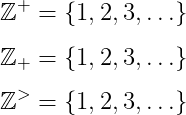## Non-negative integer symbol

The second is the non-negative integer which includes all the positive numbers including zero. And which is denoted by 0+ and symbols.

\documentclass{article}
\usepackage{amsfonts}
\begin{document}
$\mathbb{Z}^{0+}=\{0,1,2,3,\ldots\}$
$\mathbb{Z}^{\geq } =\{0,1,2,3,\ldots\}$
\end{document}

Output :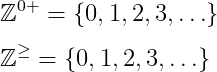## Non-zero integer symbol

And the third is the non-zero integer denoted by the and* symbols.

\documentclass{article}
\usepackage{amsfonts}
\begin{document}
$\mathbb{Z}^{\neq}=\mathbb{Z}-\{0\}$
$\mathbb{Z}^{*} =\{\dots,-2,-1,1,2,\ldots\}$
\end{document}

Output :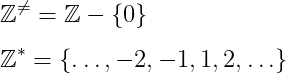Hopefully, this tutorial has been presented to you in a very simple way. Even after this, if you have any difficulty in understanding, don’t forget to comment. Thank you#### Md Jidan Mondal

LaTeX expert with over 10 years of experience in document preparation and typesetting. Specializes in creating professional documents, reports, and presentations using LaTeX.